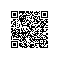# 如何用Apache Spark和LightGBM构建机器学习模型来预测信用卡欺诈

Apache Spark是一个in-memory的集群化的运行环境，用来存储和处理数据，比起从(单机）SSD或磁盘访问数据会快上千倍。假设数据集存在于Hadoop，S3，数据库或本地文件，第一步需要让Spark集群可以访问这些数据（将数据转为Spark dataframe)。在这个例子中，我将Machine Learning Group of ULB的数据，以CSV格式加载到Spark dataframe中。 Spark集群是6gb内存，部署了Databricks社区版。下面是用Spark加载数据的代码：

# File location and type
file_location = "/FileStore/tables/creditcard.csv"
file_type = "csv"

# CSV options
infer_schema = "true"
delimiter = ","

.option("inferSchema", infer_schema) \
.option("sep", delimiter) \
.load(file_location)

feature_cols = ["V" + str(i) for i in range(1,29)] + ["Amount"]
assembler = VectorAssembler(inputCols=feature_cols, outputCol="features")
stages = [assembler]

best_params = {
'bagging_fraction': 0.8,
'bagging_freq': 1,
'eval_metric': 'binary_error',
'feature_fraction': 0.944714847210862,
'lambda_l1': 1.0,
'lambda_l2': 45.0,
'learning_rate': 0.1,
'loss_function': 'binary_error',
'max_bin': 60,
'max_depth': 58,
'metric': 'binary_error',
'num_iterations': 379,
'num_leaves': 850,
'objective': 'binary',
'random_state': 7,
'verbose': None
}
lgb = LightGBMClassifier(
learningRate=0.1,
earlyStoppingRound=100,
featuresCol='features',
labelCol='label',
isUnbalance=True,
baggingFraction=best_params["bagging_fraction"],
baggingFreq=1,
featureFraction=best_params["feature_fraction"],
lambdaL1=best_params["lambda_l1"],
# lambdaL2=best_params["lambda_l2"],
maxBin=best_params["max_bin"],
maxDepth=best_params["max_depth"],
numIterations=best_params["num_iterations"],
numLeaves=best_params["num_leaves"],
objective="binary",
baggingSeed=7
)
lgb.lambdaL2, list(np.arange(1.0, 101.0, 10.0))
).build()
evaluator = BinaryClassificationEvaluator(labelCol="label",metricName="areaUnderROC")
crossValidator = CrossValidator(estimator=lgb,
estimatorParamMaps=paramGrid,
evaluator=evaluator,
numFolds=2)
stages += [crossValidator]
pipelineModel = Pipeline(stages=stages)

train, test = df.randomSplit([0.8, 0.2], seed=7)
model = pipelineModel.fit(train)
preds = model.transform(test)

binaryEvaluator = BinaryClassificationEvaluator(labelCol="label")
print ("Test Area Under ROC: " + str(binaryEvaluator.evaluate(preds, {binaryEvaluator.metricName: "areaUnderROC"})))
#True positives
tp = preds[(preds.label == 1) & (preds.prediction == 1)].count()
#True negatives
tn = preds[(preds.label == 0) & (preds.prediction == 0)].count()
#False positives
fp = preds[(preds.label == 0) & (preds.prediction == 1)].count()
#Falsel negatives
fn = preds[(preds.label == 1) & (preds.prediction == 0)].count()
print ("True Positives:", tp)
print ("True Negatives:", tn)
print ("False Positives:", fp)
print ("False Negatives:", fn)
print ("Total", preds.count())
r = float(tp)/(tp + fn)
print ("recall", r)
p = float(tp) / (tp + fp)
print ("precision", p)
f1 = 2 * p * r /(p + r)
print ("f1", f1)